Get instant live expert help with Excel or Google Sheets“My Excelchat expert helped me in less than 20 minutes, saving me what would have been 5 hours of work!”

#### Post your problem and you’ll get Expert help in seconds.

Your message must be at least 40 characters
Our professional Expert are available now. Your privacy is guaranteed.

# Flag first duplicate in a list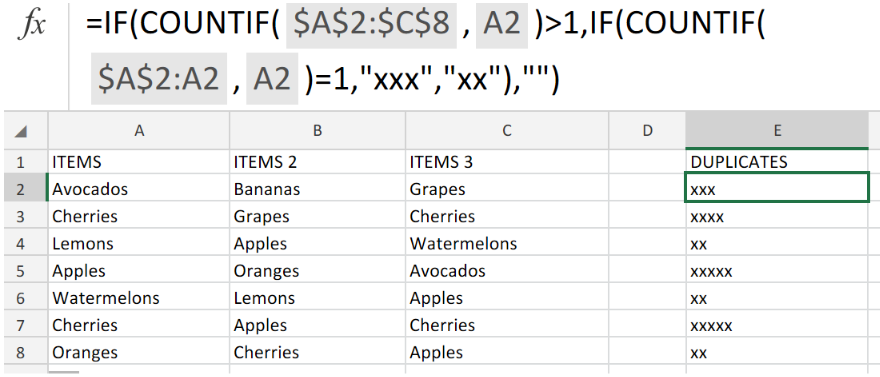Figure 1. of Flag First Duplicate in a List Excel Function.

Let’s say we are required to mark/flag the first duplicate in a list of items, we can use the COUNTIF function to achieve this. In this tutorial, we will step through the process of flagging duplicate values in a list through by nesting COUNTIF and IF functions.

## Generic Formula

`=IF(COUNTIF(A:A,A1)>1,IF(COUNTIF(A\$1:A1,A1)=1,"x","xx"),"")`

## How to use the COUNTIF Function in Excel.

Essentially, the Excel COUNTIF Function is an operation syntax enclosed by an IF statement.

We are going to illustrate its use in the following three simple steps;

1. Collect arrange data items in our worksheet.

In this example, we are going to be working with three lists of items in a grocery store. These are the items we need to check for duplicates. See illustration below;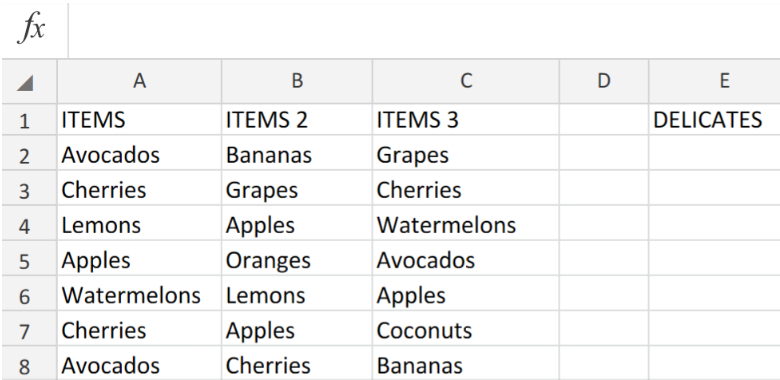Figure 2. of Items to be Checked for Duplicates.

1. In cell F2 insert this formula `=IF(COUNTIF(\$A\$2:\$C\$8,A2)>1,IF(COUNTIF(\$A\$2:A2,A2)=1,"x","xx"),"")`

This will check all of the items in columns A,B and C for any duplicated items and flag the amount of duplicates (x, xx, xxx, etc) in column E of our worksheet.

See example illustrated below;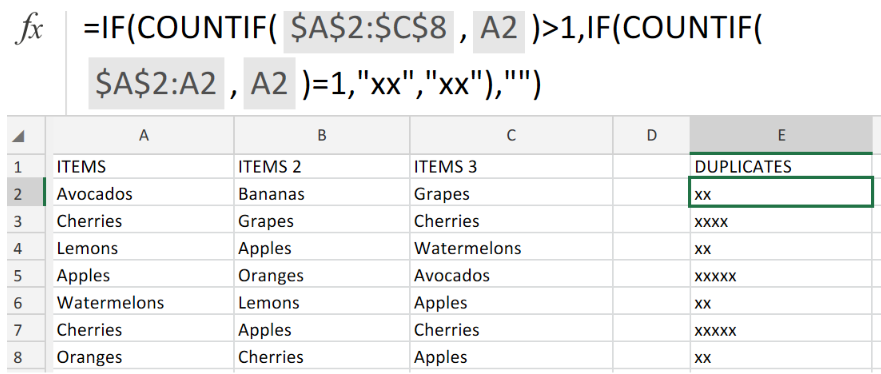Figure 3. of Flag First Duplicate in a List Excel.

1. Modify and enter the formula syntax in the example illustrated above, into the formula bar for the other cells in column F to get the desired results.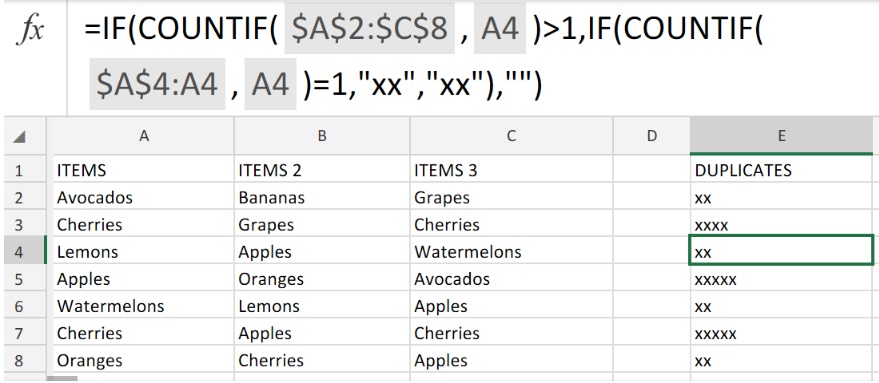Figure 4. of Flag First Duplicate in a List Excel.

## Note

The initial segment of our formula syntax consists of an expansion reference (\$A\$2:C8) which is expanding while the formula syntax is modified and entered into cells down our list columns. (The initial A2 in our cell range is locked (absolute), the next one can be altered, so it is relative  as the operation syntax is inputted down our listed items.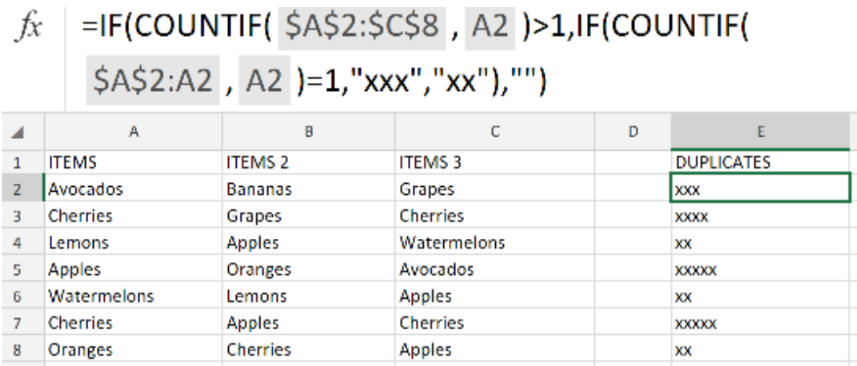Figure 5. of Final Result.

## Instant Connection to an Expert through our Excelchat Service

Our live Excelchat Service is here for you. We have Excel Experts available 24/7 to answer any Excel questions you may have. Guaranteed connection within 30 seconds and a customized solution for you within 20 minutes

### Did this post not answer your question? Get a solution from connecting with the expert.Another blog reader asked this question today on Excelchat:
Solution examplesHello, I am trying to create a total color column? Can anyone help?
Solved by I. A. in 60 minsI need a formula to check text values in a range and output one of three possible outputs into a cell. ie: If all text values in cell range = A, then Output 1. If all text values in cell range = B, then Output 2. If there is a mix of A and B values in cell range, then Output 3.
Solved by M. J. in 60 minsI need a formula to count the number of rows that contain at least one of two possible phrases, but not give me the total number of instances that both phrases occur overall.
Solved by E. B. in 33 minsHello, I have a spreadsheet with 3159 rows. One column consists of 9-digit numbers in each of the 3159 rows. I need the formula that can calculate how many of those numbers appear more than once.
Solved by D. H. in 56 minshello i need help creating a formula, if a value does not exsits put 0, if a value exist do nothing
Solved by M. F. in 9 mins## Subscribe to Excelchat.coAnother blog reader asked this question today on Excelchat: